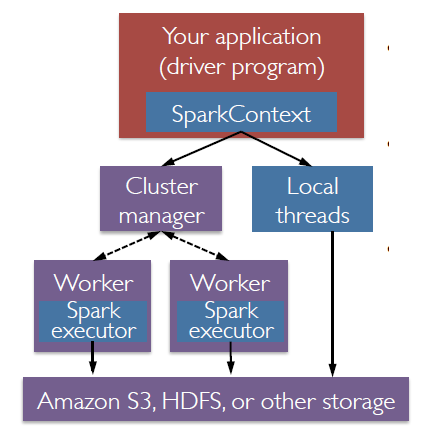# Spark - Application Execution Configuration

Computer - Capacity Planning (Sizing) in Spark to run an app

ie how to calculate:

• Num-executors - The number of concurrent tasks (executor) that can be executed.
• Executor-memory - The amount of memory allocated to each executor.
• Executor-cores - The number of cores allocated to each executor.

## Steps

### Cluster Info

via Ambari or any admin, we can get the cluster composition

For instance:

• 8 D4v2 nodes
• Memory = 8 x 25 Gb since each D4v2 has 25GB of YARN memory.
``````Total YARN memory = nodes * YARN memory per node
Total YARN memory = 8 nodes * 25GB = 200GB```
```
• How many apps are running - for instance 2 apps on your cluster, including the one you are going to run.

### Set

#### memory

For instance: 6GB of executor-memory are needed to load the data

````set  executor-memory = 6GB`
```

#### executor-cores

Setting cores per executor to larger than 4 may cause garbage collection problems.

````set executor-cores = 4`
```

#### num-executors

The num-executors parameter is determined by taking the minimum of the memory constraint and the CPU constraint divided by the # of apps running on Spark.

• Calculate memory constraint – The memory constraint is calculated as the total YARN memory divided by the memory per executor.
``````Memory constraint = (total YARN memory / executor memory) / # of apps
Memory constraint = (200GB / 6GB) / 2
Memory constraint = 16 (rounded)```
```
• Calculate CPU constraint - The CPU constraint is calculated as the total yarn cores divided by the number of cores per executor.
``````YARN cores = nodes in cluster * # of cores per node * 2
YARN cores = 8 nodes * 8 cores per D14 * 2 = 128
CPU constraint = (total YARN cores / # of cores per executor) / # of apps
CPU constraint = (128 / 4) / 2
CPU constraint = 16```
```
• Set num-executors
``````num-executors = Min (memory constraint, CPU constraint)
num-executors = Min (16, 16)
num-executors = 16```
```

## Documentation / Reference

Discover MoreSpark - Application

An application is an instance of a driver created via the initialization of a spark context (RDD) or a spark session (Data Set) This instance can be created via: a whole script (called batch mode)...